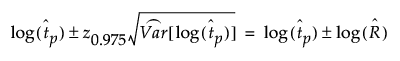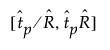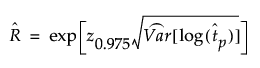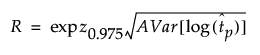Publication date: 05/24/2021

## R Precision Factor (for a 95% CI)

The R precision factor is a measure of the precision of the estimate of tp, the pth quantile of the failure time distribution. An approximate 95% confidence interval for log(tp) is:resulting in a confidence interval for tp;whereTo obtain R, use the large sample approximation of the variance of the log(tp):